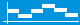# Why is the median useful

## Descriptive statistics Interpretation of data using measures of location (median, arithmetic mean, quartile, percentile)

Median

An observation or measured value is called a median if at least 50% of all characteristic values ​​of a characteristic are less than or equal to and at least 50% of all observation values ​​are greater than or equal to this median. The median is therefore exactly in the middle of a ranking list. (see: above picture)

Arithmetic means
short:
Mean or average

The arithmetic mean is calculated as the quotient of the sum of all observation values ​​divided by the number of all observation values.
The arithmetic mean is often simply called the mean or even the average.

Geometric
and
harmonic mean

In special situations the use of an arithmetic mean may not be appropriate. Thus, when comparing growth factors, the geometric mean and when comparing ratios, the harmonic mean offers a better reduction of the information.

lower and upper
Quartile

An observation value is called the upper quartile if at least 25% of all observation values ​​are greater than or equal to this upper quartile. Accordingly, an observation value is called the lower quartile if at least 25% is less than or equal to the lower quartile. (see: picture above)

lower and upper
Percentile

An observation value is called the upper percentile if at least 10% of all observation values ​​are greater than or equal to the upper percentile. Accordingly, an observation value is called the lower percentile if at least 10% of all observation values ​​are less than or equal to the lower percentile.Ideas for possible, self-organized
Exercises:

• Carries out a survey or a series of measurements. You can find suggestions for all real problems on the page: "Create a survey and evaluate it."
• Calculates and determines the measures of location that are useful for you.
You can also do calculations with the Excel tool

Examples of location dimensions